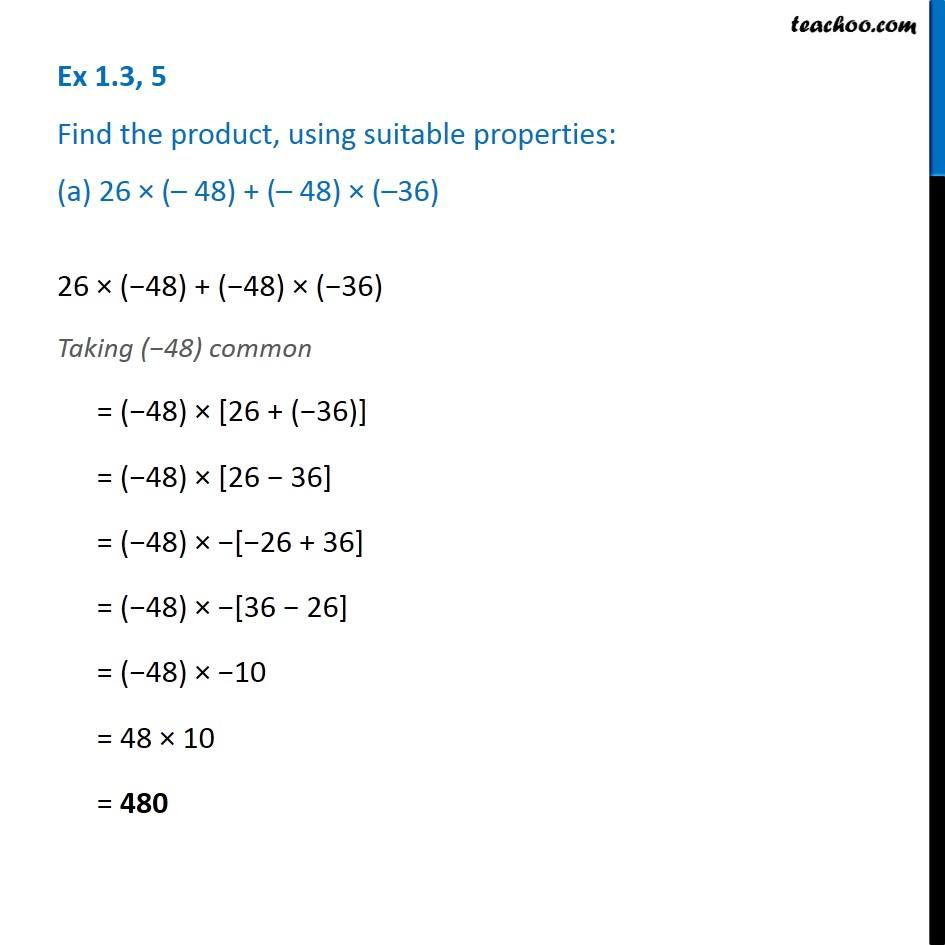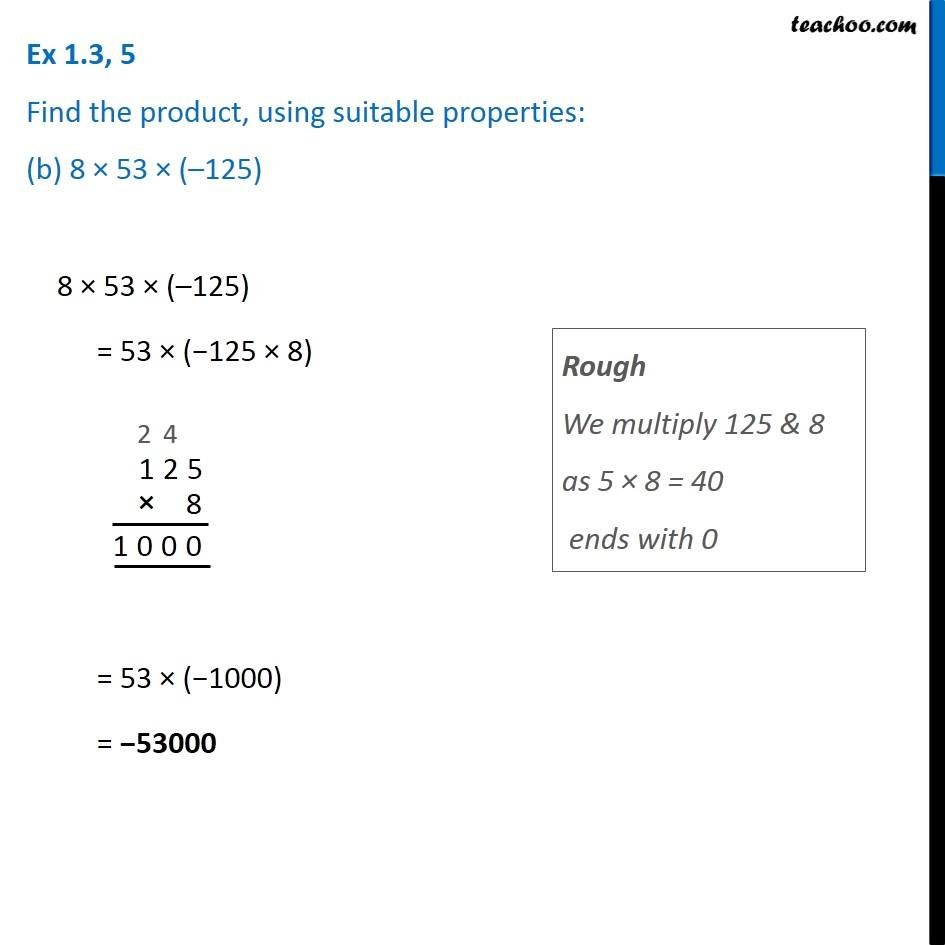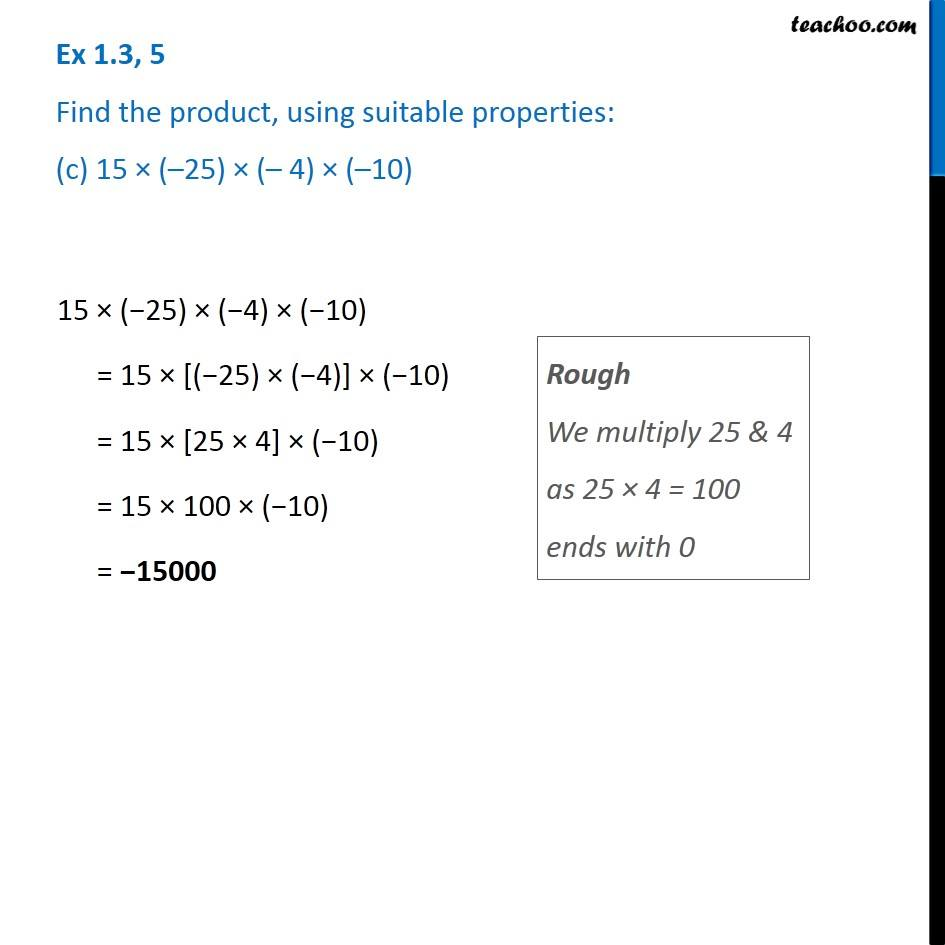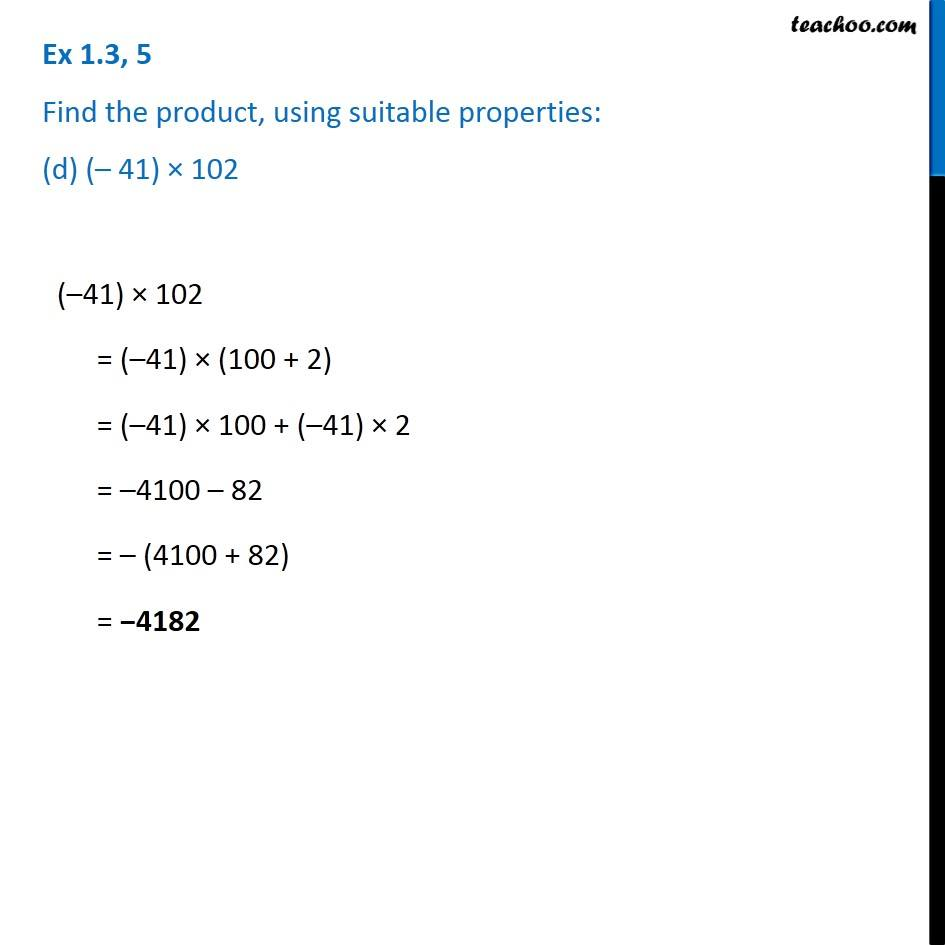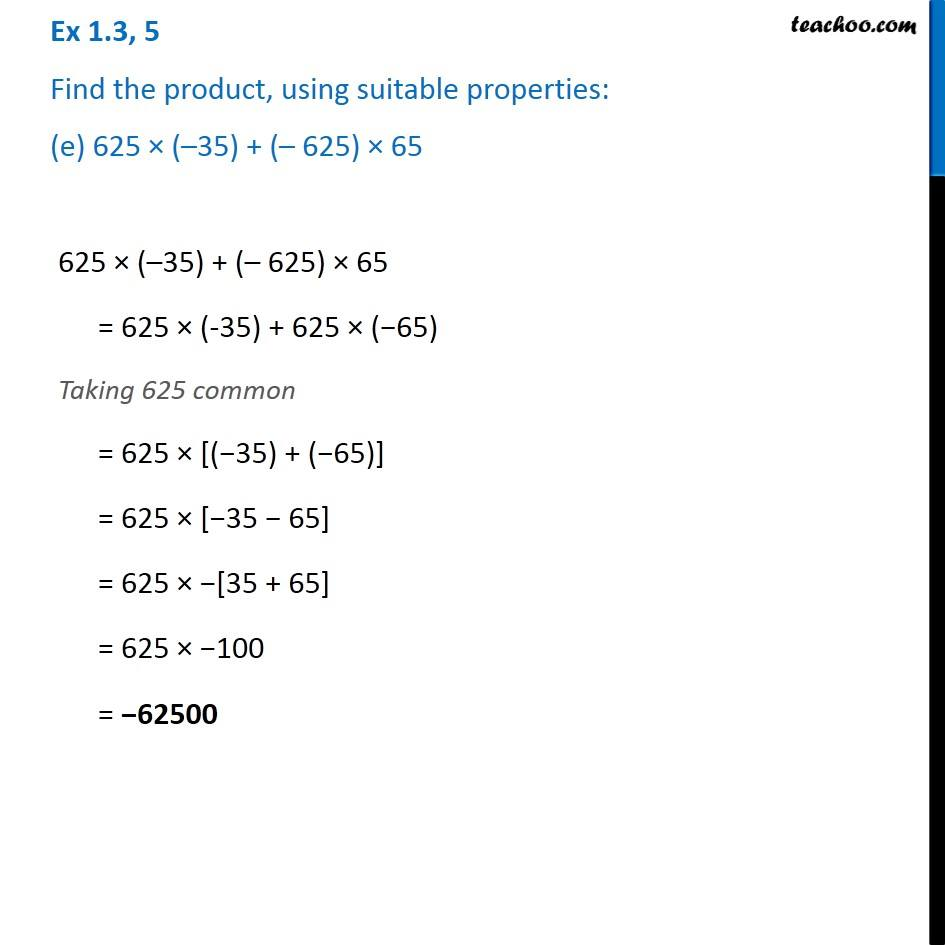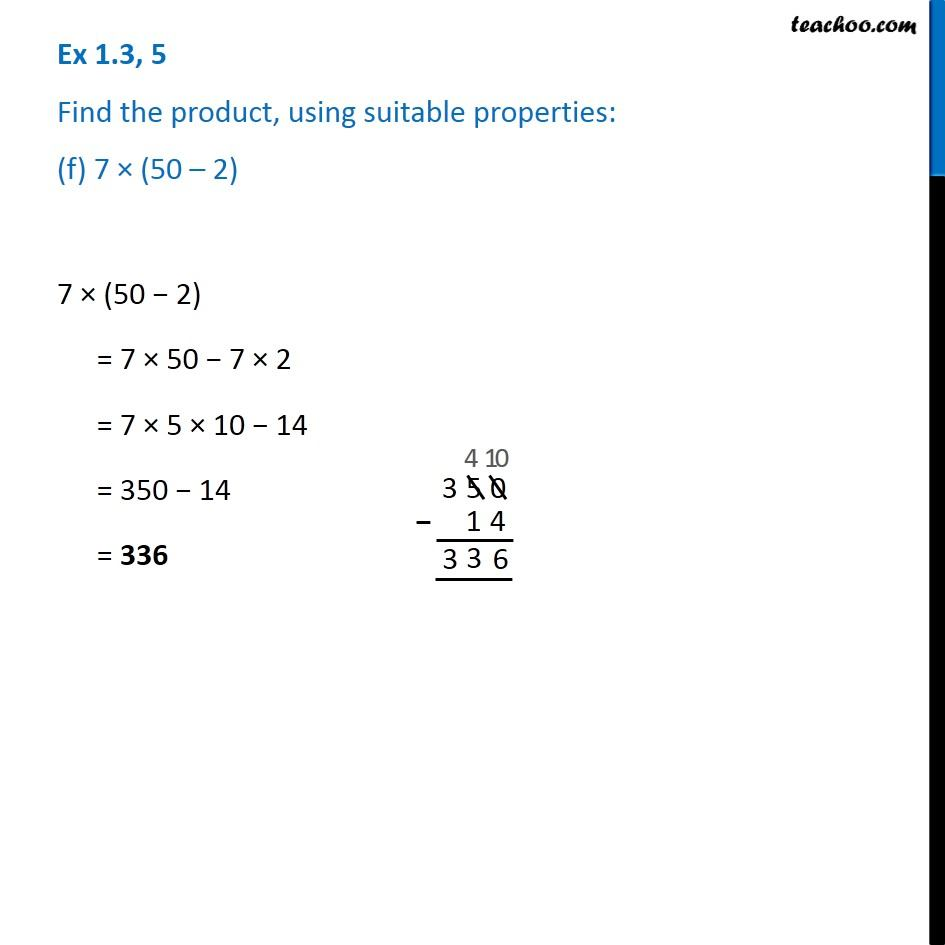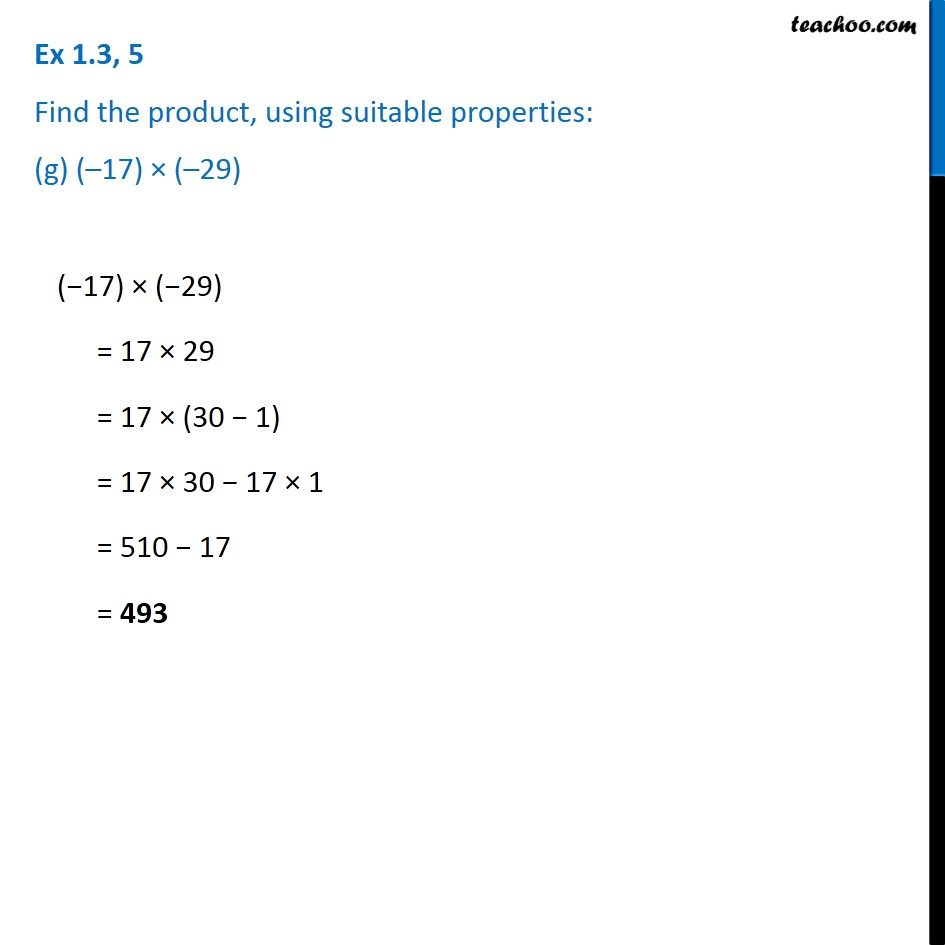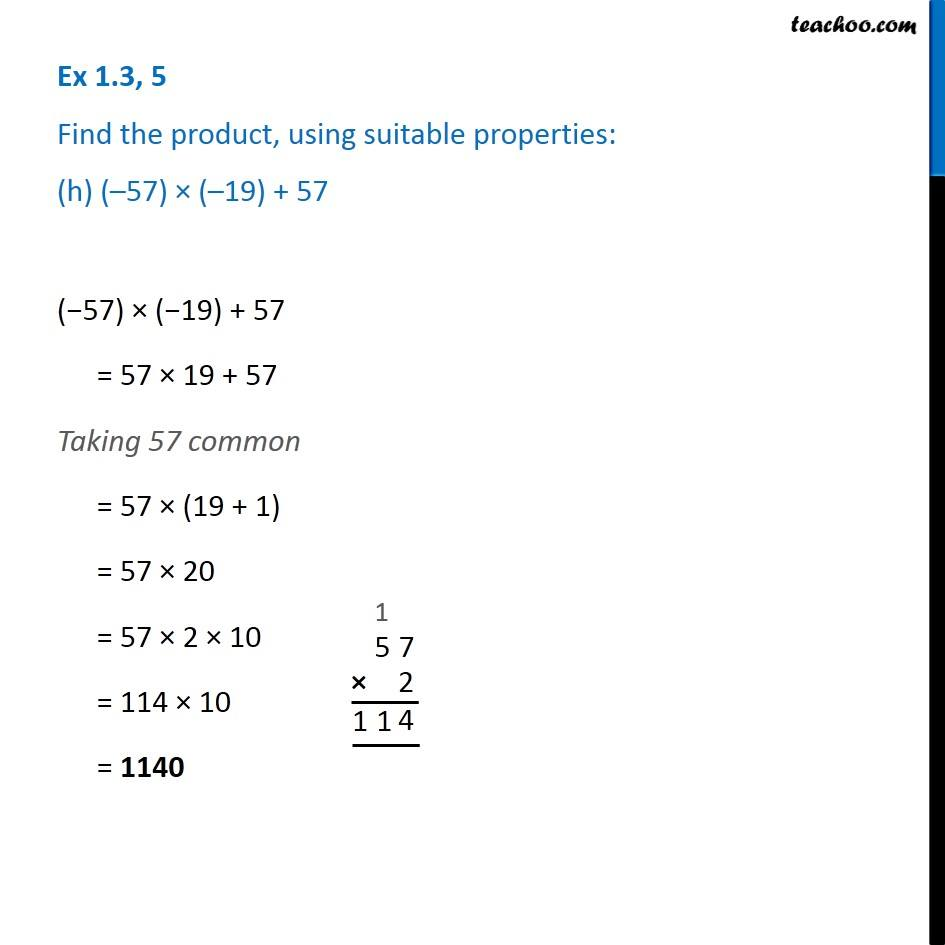Subscribe to our Youtube Channel - https://you.tube/teachoo

1. Chapter 1 Class 7 Integers
2. Serial order wise
3. Ex 1.3

Transcript

Ex 1.3, 5 Find the product, using suitable properties: (a) 26 × (– 48) + (– 48) × (–36) 26 × (−48) + (−48) × (−36) Taking (−48) common = (−48) × [26 + (−36)] = (−48) × [26 − 36] = (−48) × −[−26 + 36] = (−48) × −[36 − 26] = (−48) × −10 = 48 × 10 = 480 Ex 1.3, 5 Find the product, using suitable properties: (b) 8 × 53 × (–125) 8 × 53 × (–125) = 53 × (−125 × 8) Rough We multiply 125 & 8 as 5 × 8 = 40 ends with 0 = 53 × (−1000) = −53000 Ex 1.3, 5 Find the product, using suitable properties: (c) 15 × (–25) × (– 4) × (–10) Rough We multiply 25 & 4 as 25 × 4 = 100 ends with 0 15 × (−25) × (−4) × (−10) = 15 × [(−25) × (−4)] × (−10) = 15 × [25 × 4] × (−10) = 15 × 100 × (−10) = −15000 Ex 1.3, 5 Find the product, using suitable properties: (d) (– 41) × 102 (–41) × 102 = (–41) × (100 + 2) = (–41) × 100 + (–41) × 2 = –4100 – 82 = – (4100 + 82) = −4182 Ex 1.3, 5 Find the product, using suitable properties: (e) 625 × (–35) + (– 625) × 65 625 × (–35) + (– 625) × 65 = 625 × (-35) + 625 × (−65) Taking 625 common = 625 × [(−35) + (−65)] = 625 × [−35 − 65] = 625 × −[35 + 65] = 625 × −100 = −62500 Ex 1.3, 5 Find the product, using suitable properties: (f) 7 × (50 – 2) 7 × (50 − 2) = 7 × 50 − 7 × 2 = 7 × 5 × 10 − 14 = 350 − 14 = 336 Ex 1.3, 5 Find the product, using suitable properties: (g) (–17) × (–29) (−17) × (−29) = 17 × 29 = 17 × (30 − 1) = 17 × 30 − 17 × 1 = 510 − 17 = 493 Ex 1.3, 5 Find the product, using suitable properties: (h) (–57) × (–19) + 57 (−57) × (−19) + 57 = 57 × 19 + 57 Taking 57 common = 57 × (19 + 1) = 57 × 20 = 57 × 2 × 10 = 114 × 10 = 1140

Ex 1.3

Chapter 1 Class 7 Integers
Serial order wise